# Tangent-cubed function

This article is about a particular function from a subset of the real numbers to the real numbers. Information about the function, including its domain, range, and key data relating to graphing, differentiation, and integration, is presented in the article.
View a complete list of particular functions on this wiki
For functions involving angles (trigonometric functions, inverse trigonometric functions, etc.) we follow the convention that all angles are measured in radians. Thus, for instance, the angle of$90\,^\circ$ is measured as$\pi/2$.

## Definition

This function, denoted$\tan^3$, is defined as the composite of the cube function and the tangent function.

## Differentiation

### First derivative

To calculate the first derivative of$\tan^3$, we note that the function is the composite of the cube function and the tangent function, and differentiate using the chain rule for differentiation: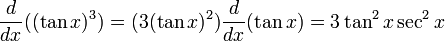$\frac{d}{dx}((\tan x)^3) = (3(\tan x)^2)\frac{d}{dx}(\tan x) = 3\tan^2x \sec^2x$

### Higher derivatives

Fill this in later

## Integration

### First antiderivative

We use the identity: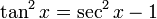$\! \tan^2x = \sec^2x - 1$

as follows: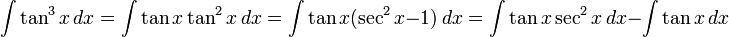$\int \tan^3x \, dx = \int \tan x \tan^2x \, dx = \int \tan x (\sec^2x - 1) \, dx = \int \tan x \sec^2x \, dx - \int \tan x \, dx$

The term$\tan x \sec^2x$ integrates to$(\tan^2x)/2$ (we can see this, for instance, by putting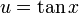$u = \tan x$, see also tan.sec^2#Integration). The term$\tan x$ integrates to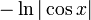$-\ln|\cos x|$ (See tan#Integration). We thus get:$\frac{\tan^2x}{2} + \ln|\cos x| + C$

Note that the value of$C$ is constant within every connected component of the domain, but could differ between different connected components of the domain.

### Higher antiderivatives

It is not possible to integrate this function repeatedly in terms of elementary functions, because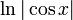$\ln|\cos x|$ cannot be integrated in terms of elementary functions.